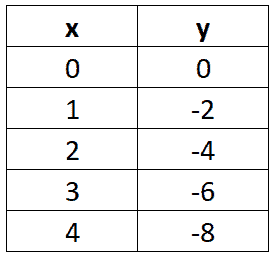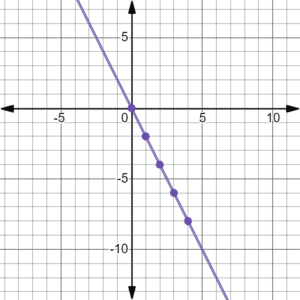This video shows how patterns in function charts give you different values. Linear functions are where the function output changes at a constant rate, relative to the input. After you finish this lesson, view all of our Algebra 1 lessons and practice problems.

An example of this would be the function:$y = 4x$$y = 4(1) = 4$$y = 4(2) = 8$$y = 4(3) = 12$
This function increases at a constant rate, as the input increases or decreases at a constant rate.

Example of Patterns And Linear Functions

Example 1The linear function would be:$y=2x+1$

Example 2The linear function would be:$y=2x$

Video-Lesson Transcript

Let’s go over patterns and linear functions.

Think of a linear function as a shape. When you put something in, it comes out as something else.

For example, when you put$x$ in a linear function, it will come out as$y$.

For example when we have$x = 0$ our$y = 3$. If$x = 2$,$y = 9$ and if$x = 7$,$y = 28$.

So how does this relates to linear functions?

When we graph using$x$ and$y$ axis and we plot the$x$-coordinates and$y$-coordinates, we will form a straight line. That’s something special about the linear function.

Here’s an example of a linear function:

When$x = 0$,$y = 3$,
when$x = 1$,$y = 5$,
if$x = 2$,$y = 7$,
when$x = 3$,$y = 9$,
and when$x = 4$,$y = 11$.

What makes this linear just by looking at this?

It’s because it increases at a constant rate.

When$x$ increase by$1$,$y$ increase by$2$.

Another example:

When$x = 3$,$y = 15$,
when$x = 4$,$y = 19$,
if$x = 5$,$y = 23$,
when$x = 6$,$y = 27$,
and when$x = 7$,$y = 31$.

Here when$x$ increase by$1$,$y$ increase by$4$.

Now, let’s graph this.

You will see that it’ll give us a straight slash line.Let’s have another example:

When$x = 0$,$y = 0$,
when$x = 1$,$y = 3$,
if$x = 2$,$y = 6$,
when$x = 3$,$y = 9$,
and when$x = 4$,$y = 12$

Here, its$y = 3x$.

Another example is:

When$x = 0$,$y = 0$,
if$x = 1$,$y = 4$,
when$x = 2$,$y = 8$,
and when$x = 3$,$y = 12$

Here,$y = 4x$.

So we have$y = 4x$$y = 4(0) = 0$$y = 4(1) = 4$$y = 4(2) = 8$$y = 4(3) = 12$

Same thing with the example above.

But they don’t always work out this way.

Let’s have a more complicated example.

When$x = 0$,$y = 2$,
when$x = 1$,$y = 5$,
if$x = 2$,$y = 8$,
when$x = 3$,$y = 11$,
and when$x = 4$,$y = 14$

It’s not like our previous examples where it’s a simple equation.

Here, we’re going to have a$y = 3x + 2$

Let’s check using$x = 4$, let’s substitute$y = 3x + 2$$y = 3(4) + 2$$y = 12 + 2$$y = 14$